# High School Math : How to find the perimeter of an equilateral triangle

## Example Questions

### Example Question #1 : How To Find The Perimeter Of An Equilateral Triangle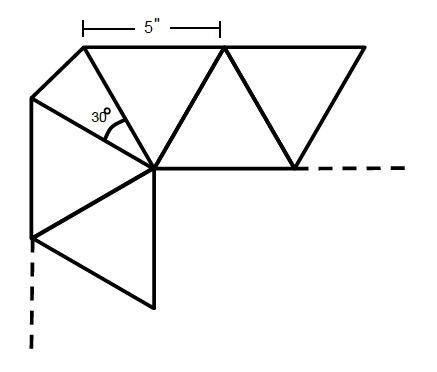A square rug border consists of a continuous pattern of equilateral triangles, with isosceles triangles as corners, one of which is shown above. If the length of each equilateral triangle side is 5 inches, and there are 40 triangles in total, what is the total perimeter of the rug?

The inner angles of the corner triangles is 30°.

124

180

208

200

188

188

Explanation:

There are 2 components to this problem. The first, and easier one, is recognizing how much of the perimeter the equilateral triangles take up—since there are 40 triangles in total, there must be 40 – 4 = 36 of these triangles. By observation, each contributes only 1 side to the overall perimeter, thus we can simply multiply 36(5) = 180" contribution.

The second component is the corner triangles—recognizing that the congruent sides are adjacent to the 5-inch equilateral triangles, and the congruent angles can be found by

180 = 30+2x → x = 75°

We can use ratios to find the unknown side:

75/5 = 30/y → 75y = 150 → y = 2''.

Since there are 4 corners to the square rug, 2(4) = 8'' contribution to the total perimeter. Adding the 2 components, we get 180+8 = 188 inch perimeter.

### Example Question #2 : How To Find The Perimeter Of An Equilateral Triangle

The height of an equilateral triangle is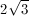What is the triangle's perimeter?

8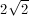6

24

12

12

Explanation:

An altitude drawn in an equilateral triangle will form two 30-60-90 triangles. The height of equilateral triangle is the length of the longer leg of the 30-60-90 triangle. The length of the equilateral triangle's side is the length of the hypotenuse of the 30-60-90.

The ratio of the length of the hypotenuse to the length of the longer leg of a 30-60-90 triangle is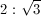The length of the longer leg of the 30-60-90 triangle in this problem isUsing this ratio, we find that the length of this triangle's hypotenuse is 4. Thus the perimeter of the equilateral triangle will be 4 multiplied by 3, which is 12.

### Example Question #3 : How To Find The Perimeter Of An Equilateral Triangle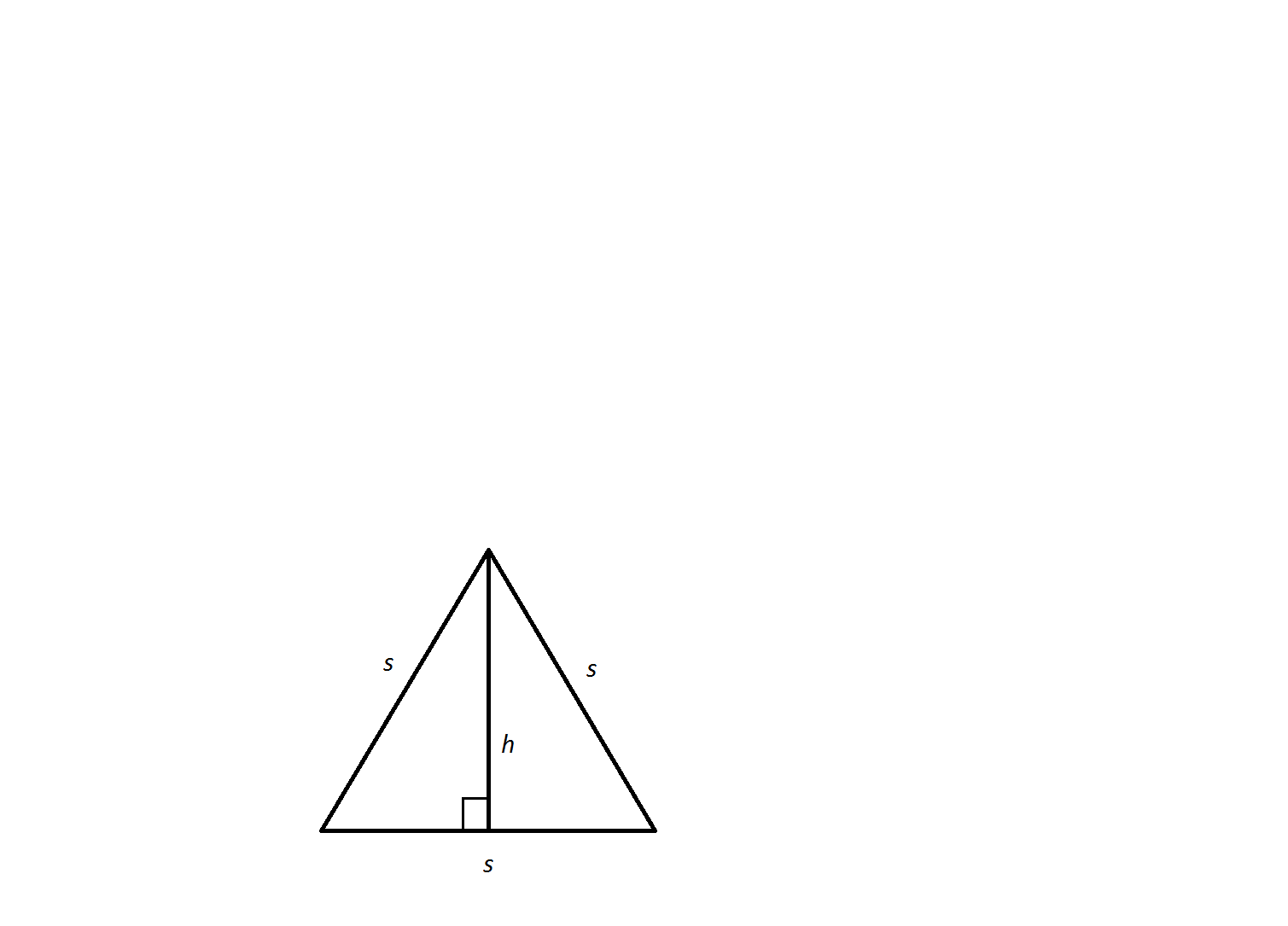An equilateral triangle has a side length of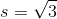.  What is its perimeter?

Not enough information to solve.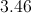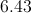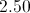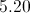Explanation:

An equilateral triangle possesses three sides of equal lengths.  Therefore, we can easily calculate its perimeter by tripling the given side length.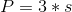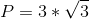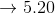### Example Question #4 : How To Find The Perimeter Of An Equilateral TriangleAn equilateral triangle has an altitude length of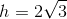.  What is its perimeter?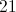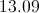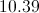Not enough information to solveExplanation:

An altitude slices an equilateral triangle into two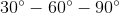triangles. These triangles follow a side length pattern. The smallest of the two legs equalsand the hypotenuse equals. By way of the Pythagorean Theorem, the longest leg or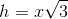.

We have the length of the altitude of the triangle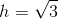. We can solve for the smallest sidevia substitution and simple algebra.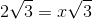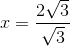Note, this is only the smallest side of one of thetriangles. It needs to be doubled to equal a complete side of the equilateral triangle.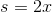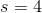Now, the side length can be tripled to calculate the perimeter.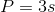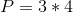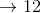### Example Question #5 : How To Find The Perimeter Of An Equilateral Triangle

Find the perimeter of the following equilateral triangle: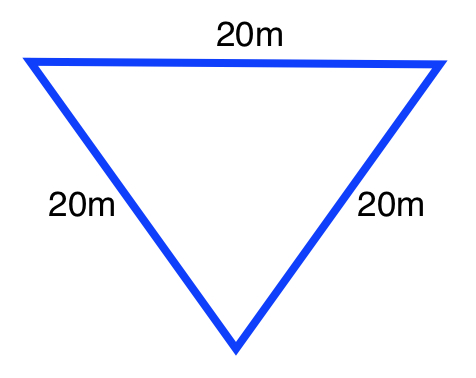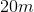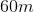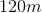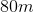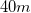Explanation:

The formula for the perimeter of an equilateral triangle is: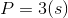Whereis the length of the side

Plugging in our values, we get: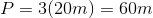### Example Question #6 : How To Find The Perimeter Of An Equilateral Triangle

Determine the perimeter of the following equilateral triangle: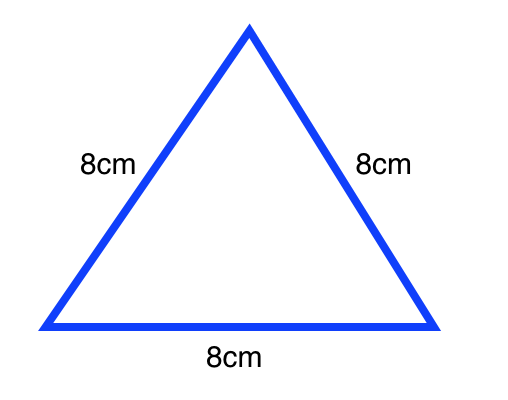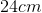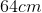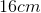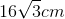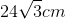Explanation:

The formula for the perimeter of an equilateral triangle is:,

whereis the length of the side.

Plugging in our value, we get: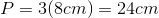### Example Question #7 : How To Find The Perimeter Of An Equilateral Triangle

An equilateral triangle has a side of lengthfeet. What is the perimeter of the triangle?feet

It cannot be determined from the information given.feetfootfeetfeet
An equilateral triangle by definition. has three congruent sides. Thus, if one side isfeet long, then all three sides are eachfeet long. We also know that the perimeter is the sum of all sides. Therefore, our perimeter is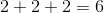Since we were given units of feet, our final answer isfeet.